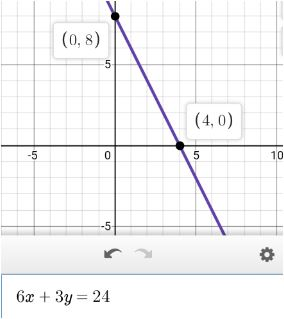# Assume that it takes six hours of labor time to paint a room and three hours to sand a floor. If...

## Question:

Assume that it takes six hours of labor time to paint a room and three hours to sand a floor. If {eq}24 {/eq} hours were spent painting

(a) How many rooms could be painted by one worker?

(b) If a decision were made to sand two floors, how many painted rooms would have to be given up?

(c) Illustrate with a production-possibilities curve.

## Linear Equation in two variables:

This problem involves understanding a given linear equation in two variables to find the answers to the following subparts. First, we need to generate a linear equation in two variables. Later, we have drawn a curve of a number of rooms painted vs the number of floors sanded curve which gives us the idea about production-possibility.

Given, it takes six hours of labor time to paint a room and three hours to sand a floor.

(a) If 24 hours were spent painting a room, a total of 4 rooms would have been painted.

(b) If a decision was made to sand two floors, so the time spent on painting = 24 - 2(3) = 18

Thus, 3 rooms would be painted. Hence, one painted room had to be given up.

(c) The equation will be like -

{eq}\displaystyle 6x + 3y = 24 {/eq}

Where the x-axis is the number of rooms and the y-axis represents the number of floors that were sanded. The curve will look something like -